### IMO Shortlist 1984 problem 6

Kvaliteta:
Avg: 0,0
Težina:
Avg: 0,0
Let$c$ be a positive integer. The sequence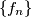$\{f_n\}$ is defined as follows: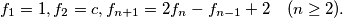Show that for each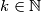$k \in \mathbb N$ there exists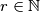$r \in \mathbb N$ such that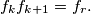$f_kf_{k+1}= f_r.$
Izvor: Međunarodna matematička olimpijada, shortlist 1984